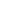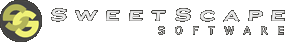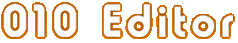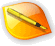Edit AnythingProfessional text and hex editingwith Binary Templates technology.010 Editor - Text/Hex Editor Homepage

 Tool FunctionsThe following is a list of functions that allow running many of the tools in the Tools Menu or Search Menu, as well as functions for working with drives and processes.int64 Checksum(
int algorithm,
int64 start=0,
int64 size=0,
int64 crcPolynomial=-1,
int64 crcInitValue=-1 )

Runs a simple checksum on a file and returns the result as a int64. The algorithm can be one of the following constants:

• CHECKSUM_BYTE - Treats the file as a set of unsigned bytes
• CHECKSUM_SHORT_LE - Treats the file as a set of unsigned little-endian shorts
• CHECKSUM_SHORT_BE - Treats the file as a set of unsigned big-endian shorts
• CHECKSUM_INT_LE - Treats the file as a set of unsigned little-endian ints
• CHECKSUM_INT_BE - Treats the file as a set of unsigned big-endian ints
• CHECKSUM_INT64_LE - Treats the file as a set of unsigned little-endian int64s
• CHECKSUM_INT64_BE - Treats the file as a set of unsigned big-endian int64s
• CHECKSUM_SUM8 - Same as CHECKSUM_BYTE except result output as 8-bits
• CHECKSUM_SUM16 - Same as CHECKSUM_BYTE except result output as 16-bits
• CHECKSUM_SUM32 - Same as CHECKSUM_BYTE except result output as 32-bits
• CHECKSUM_SUM64 - Same as CHECKSUM_BYTE
• CHECKSUM_CRC16
• CHECKSUM_CRCCCITT
• CHECKSUM_CRC32

If start and size are zero, the algorithm is run on the whole file. If they are not zero then the algorithm is run on size bytes starting at local address start. See the ChecksumAlgBytes and ChecksumAlgStr functions to run more complex algorithms. crcPolynomial and crcInitValue can be used to set a custom polynomial and initial value for the CRC functions. A value of -1 for these parameters uses the default values as described in the Check Sum/Hash Algorithms topic. A negative number is returned on error.int ChecksumAlgArrayStr(
int algorithm,
char result[],
uchar *buffer,
int64 size,
char ignore[]="",
int64 crcPolynomial=-1,
int64 crcInitValue=-1 )

Similar to the ChecksumAlgStr function except that the checksum is run on data stored in an array instead of in a file. The data for the checksum should be passed in the buffer array and the size parameter lists the number of bytes in the array. The result from the checksum will be stored in the result string and the number of characters in the string will be returned, or -1 if an error occurred. See the ChecksumAlgStr function for a list of available algorithms.

Requires 010 Editor v4.0 or higher.int ChecksumAlgArrayBytes(
int algorithm,
uchar result[],
uchar *buffer,
int64 size,
char ignore[]="",
int64 crcPolynomial=-1,
int64 crcInitValue=-1 )

Similar to the ChecksumAlgStr function except that the checksum is run on data in an array instead of in a file and the results are stored in an array of bytes instead of a string. The data for the checksum should be passed in the buffer array and the size parameter lists the number of bytes in the array. The result of the checksum operation will be stored as a set of hex bytes in the parameter result. The function will return the number of bytes placed in the result array or -1 if an error occurred. See the ChecksumAlgStr function for a list of available algorithms.

Requires 010 Editor v4.0 or higher.int ChecksumAlgStr(
int algorithm,
char result[],
int64 start=0,
int64 size=0,
char ignore[]="",
int64 crcPolynomial=-1,
int64 crcInitValue=-1 )

Similar to the Checksum algorithm except the following algorithm constants are supported:

• CHECKSUM_BYTE
• CHECKSUM_SHORT_LE
• CHECKSUM_SHORT_BE
• CHECKSUM_INT_LE
• CHECKSUM_INT_BE
• CHECKSUM_INT64_LE
• CHECKSUM_INT64_BE
• CHECKSUM_SUM8
• CHECKSUM_SUM16
• CHECKSUM_SUM32
• CHECKSUM_SUM64
• CHECKSUM_CRC16
• CHECKSUM_CRCCCITT
• CHECKSUM_CRC32
• CHECKSUM_MD2
• CHECKSUM_MD4
• CHECKSUM_MD5
• CHECKSUM_RIPEMD160
• CHECKSUM_SHA1
• CHECKSUM_SHA256
• CHECKSUM_SHA384
• CHECKSUM_SHA512
• CHECKSUM_TIGER

The result argument specifies a string which will hold the result of the checksum. The return value indicates the number of characters in the string, or is negative if an error occurred. Any ranges to ignore can be specified in string format with the ignore argument (see Check Sum/Hash Algorithms). The crcPolynomial and crcInitValue parameters are used to set a custom polynomial and initial value for the CRC algorithms. Specifying -1 for these parameters uses the default values as indicated in the Check Sum/Hash Algorithms help topic. See the Checksum function above for an explanation of the different checksum constants.

Requires 010 Editor v12.0 or higher for CHECKSUM_SHA384.int ChecksumAlgBytes(
int algorithm,
uchar result[],
int64 start=0,
int64 size=0,
char ignore[]="",
int64 crcPolynomial=-1,
int64 crcInitValue=-1 )

This function is identical to the ChecksumAlgStr function except that the checksum is returned as a byte array in the result argument. The return value is the number of bytes returned in the array.TCompareResults Compare(
int type,
int fileNumA,
int fileNumB,
int64 startA=0,
int64 sizeA=0,
int64 startB=0,
int64 sizeB=0,
int matchcase=true,
int64 minmatchlength=8,
int64 quickmatch=512 )

Runs a comparison between two files or between two blocks of data. The type argument indicates the type of comparison that should be run and can be either:

• COMPARE_SYNCHRONIZE (a binary comparison)
• COMPARE_SIMPLE (a byte-by-byte comparison)

fileNumA and fileNumB indicate the numbers of the file to compare (see GetFileNum). The file numbers may be the same to compare two blocks in the same file. The startA, sizeA, startB, and sizeB arguments indicate the size of the blocks to compare in the two files. If the start and size are both zero, the whole file is used. If matchcase is false, then letters of mixed upper and lower cases will match. See Comparing Files for details on the maxlookahead, minmatchlength and quickmatch arguments. The return value is TCompareResults structure with contains a count variable indicating the number of resulting ranges, and an array of record. Each record contains the variables type, startA, sizeA, startB, and sizeB to indicate the range. The type variable will be one of:

• COMPARE_MATCH=0
• COMPARE_DIFFERENCE=1
• COMPARE_ONLY_IN_A=2
• COMPARE_ONLY_IN_B=3

For example:

```    int i, f1, f2;
FileOpen( "C:\\temp\\test1" );
f1 = GetFileNum();
FileOpen( "C:\\temp\\test2" );
f2 = GetFileNum();
TCompareResults r = Compare( COMPARE_SYNCHRONIZE, f1, f2 );
for( i = 0; i < r.count; i++ )
{
Printf( "%d %Ld %Ld %Ld %Ld\n",
r.record[i].type,
r.record[i].startA,
r.record[i].sizeA,
r.record[i].startB,
r.record[i].sizeB );
}
```

Note that startA and startB are specified in local coordinates.char ConvertASCIIToEBCDIC( char ascii )
Converts the given ASCII character into an EBCDIC character and returns the result.void ConvertASCIIToUNICODE(
int len,
const char ascii[],
ubyte unicode[],
int bigendian=false )

Converts an ASCII string into an array of bytes and stores them in the unicode argument. len indicates the number of characters to convert and the unicode array must be of size at least 2*len. If bigendian is true, the bytes are stored in big-endian mode, otherwise the bytes are stored in little-endian mode.void ConvertASCIIToUNICODEW(
int len,
const char ascii[],
ushort unicode[] )

Converts an ASCII string into an array of words and stores the array in the unicode argument. The number of characters to convert is given by the len argument and the unicode argument must have size at least len.char ConvertEBCDICToASCII( char ebcdic )
Converts the given EBCDIC character into an ASCII character and returns the result.void ConvertUNICODEToASCII(
int len,
const ubyte unicode[],
char ascii[],
int bigendian=false )

Converts an array of UNICODE characters in the unicode argument into ASCII bytes and stores them in the ascii array. len indicates the number of characters to convert. unicode must be of size at least size 2*len and ascii must be of size at least len. If bigendian is true, the bytes are stored in big-endian mode, otherwise the bytes are stored in little-endian mode.void ConvertUNICODEToASCIIW(
int len,
const ushort unicode[],
char ascii[] )

Converts the array of words in the unicode argument to ASCII bytes and saves them to the ascii argument. The number of characters to convert is given by len. unicode and ascii must be of size at least size len.int ExportFile(
int type,
char filename[],
int64 start=0,
int64 size=0,
int bytesperrow=16,

Exports the currently open file to a file on disk given by filename using one of the following type formats:

• EXPORT_HEXTEXT
• EXPORT_DECTEXT
• EXPORT_BINARYTEXT
• EXPORT_CCODE
• EXPORT_JAVACODE
• EXPORT_PYTHONCODE
• EXPORT_INTEL8
• EXPORT_INTEL16
• EXPORT_INTEL32
• EXPORT_S19
• EXPORT_S28
• EXPORT_S37
• EXPORT_TEXT_AREA
• EXPORT_HTML
• EXPORT_RTF
• EXPORT_BASE64
• EXPORT_UUENCODE

The start and size arguments indicate what portion of the file to export. If they are both zero then the whole file is exported. startaddress indicates the starting file address that is written to the file for Intel Hex or Motorola formats. bytesperrow indicates the number of bytes written on each line of the output file. If wordaddresses is true and the export format is Intel Hex, the file will be written using word-based addresses. See Importing/Exporting Files for more information on exporting.

Requires 010 Editor v13.0 or higher for the EXPORT_PYTHONCODE option.TFindResults FindAll(
<datatype> data,
int matchcase=true,
int wholeword=false,
int method=0,
double tolerance=0.0,
int dir=1,
int64 start=0,
int64 size=0,
int wildcardMatchLength=24 )

This function converts the argument data into a set of hex bytes and then searches the current file for all occurrences of those bytes. data may be any of the basic types or an array of one of the types. If data is an array of signed bytes, it is assumed to be a null-terminated string. To search for an array of hex bytes, create an unsigned char array and fill it with the target value. If the type being search for is a string, the matchcase and wholeworld arguments can be used to control the search (see Using Find for more information). method controls which search method is used from the following options:

• FINDMETHOD_NORMAL=0 - a normal search
• FINDMETHOD_WILDCARDS=1 - when searching for strings use wildcards '*' or '?'
• FINDMETHOD_REGEX=2 - when searching for strings use Regular Expressions

wildcardMatchLength indicates the maximum number of characters a '*' can match when searching using wildcards. If the target is a float or double, the tolerance argument indicates that values that are only off by the tolerance value still match. If dir is 1 the find direction is down and if dir is 0 the find direction is up. start and size can be used to limit the area of the file that is searched. start is the starting local address in the file where the search will begin and size is the number of bytes after start that will be searched. If size is zero, the file will be searched from start to the end of the file.

The return value is a TFindResults structure. This structure contains a count variable indicating the number of matches, and a start array holding an array of starting positions in local coordinates, plus a size array which holds an array of target lengths. For example, use the following code to find all occurrences of the ASCII string "Test" in a file:

```    int i;
TFindResults r = FindAll( "Test" );
Printf( "%d\n", r.count );
for( i = 0; i < r.count; i++ )
Printf( "%Ld %Ld\n", r.start[i], r.size[i] );
```

To search for the floating point value 4.25 with tolerance 0.01, use the following code:

```     float value = 4.25f;
TFindResults r = FindAll( value, true, false, 0, 0.01 );
```

Type Specifiers can be used in a string as an alternate way to specify the type of data to find. For example, the string "15.5,lf" can be used to search for the double 15.5. Using type specifiers is currently the only way to search for hex bytes with wildcards. For example:

```     TFindResults r = FindAll( "FF*6A,h", true, false,
FINDMETHOD_WILDCARDS );
```

When searching for regular expressions with FindAll, remember to use '\\' to denote a backslash character. For example, to find all numbers with 8 digits use:

```     TFindResults r = FindAll( "\\b\\d{8}\\b", true, false,
FINDMETHOD_REGEX );
```
Requires 010 Editor v4.0 or higher for the wildcardMatchLength parameter.
Requires 010 Editor v6.0 or higher for method=FINDMETHOD_REGEX.int64 FindFirst(
<datatype> data,
int matchcase=true,
int wholeword=false,
int method=0,
double tolerance=0.0,
int dir=1,
int64 start=0,
int64 size=0,
int wildcardMatchLength=24 )

This function is identical to the FindAll function except that the return value is the position of the first occurrence of the target found in local coordinates. A negative number is returned if the value could not be found.

Requires 010 Editor v4.0 or higher for the wildcardMatchLength parameter.
Requires 010 Editor v6.0 or higher for method=FINDMETHOD_REGEX.TFindInFilesResults FindInFiles(
<datatype> data,
char dir[],
int subdirs=true,
int openfiles=false,
int matchcase=true,
int wholeword=false,
int method=0,
double tolerance=0.0,
int wildcardMatchLength=24,

Searches for a given set of data across multiple files. See the FindAll function for information on the data, matchcase, wholeword, method, wildcardMatchLength and tolerance arguments. The dir argument indicates the starting directory where the search will take place. mask indicates which file types to search and may contain the characters '*' and '?'. If subdirs is true, all subdirectories are recursively searched for the value as well. If openfiles is true, only the currently open files are searched. If followSymbolicLinks and subdirs are true then all subdirectories that are symbolic links are searched, and if followSymbolicLinks is false then directories that are symbolic links are ignored. The return value is the TFindInFilesResults structure which contains a count variable indicate the number of files found plus an array of file variables. Each file variable contains a count variable indicating the number of matches, plus an array of start and size variables indicating the match position. For example:

```    int i, j;
TFindInFilesResults r = FindInFiles( "PK",
"C:\\temp", "*.zip" );
Printf( "%d\n", r.count );
for( i = 0; i < r.count; i++ )
{
Printf( "   %s\n", r.file[i].filename );
Printf( "   %d\n", r.file[i].count );
for( j = 0; j < r.file[i].count; j++ )
Printf( "       %Ld %Ld\n",
r.file[i].start[j],
r.file[i].size[j] );
}
```

Requires 010 Editor v4.0 or higher for the wildcardMatchLength parameter.
Requires 010 Editor v6.0 or higher for method=FINDMETHOD_REGEX.
Requires 010 Editor v11.0 or higher for followSymbolicLinks.int64 FindNext( int dir=1 )
This function returns the position of the next occurrence of the target value specified with the FindFirst function. If dir is 1, the find direction is down. If dir is 0, the find direction is up. The return value is the address of the found data, or -1 if the target is not found.TFindStringsResults FindStrings(
int minStringLength,
int type,
int matchingCharTypes,
wstring customChars="",
int64 start=0,
int64 size=0,
int requireNull=false )

Attempts to locate any strings within a binary file similar to the Find Strings dialog which is accessed by clicking 'Search > Find Strings' on the main menu. Specify the minimum length of each string in number of characters with the minStringLength parameter. The type option tells the algorithm to look for ASCII strings, UNICODE strings or both by using one of the following constants:

• FINDSTRING_ASCII
• FINDSTRING_UNICODE
• FINDSTRING_BOTH

To specify which characters are considered as part of a string, use an OR bitmask ('|') of one or more of the following constants:

• FINDSTRING_LETTERS - the letters A..Z and a..z
• FINDSTRING_LETTERS_ALL - all international numbers including FINDSTRING_LETTERS
• FINDSTRING_NUMBERS - the numbers 0..9
• FINDSTRING_NUMBERS_ALL - all international numbers including FINDSTRING_NUMBERS
• FINDSTRING_SYMBOLS - symbols such as '#', '@', '!', etc. except for '_'
• FINDSTRING_UNDERSCORE - the character '_'
• FINDSTRING_SPACES - spaces or whitespace
• FINDSTRING_LINEFEEDS - line feed characters 0x0a, 0x0d
• FINDSTRING_CUSTOM - include any custom characters in the customChars string

Note if the FINDSTRING_CUSTOM constant is included, any characters from customChars are considered as part of the string otherwise the customChars string is ignored. The start and size parameters indicate the range of the file to search and if size is zero, the file is searched starting from local address start to the end of the file. If requireNull is true, the strings must have a null (0) character after each string.

The return value is a TFindStringsResults structure which contains a count variable with the number of strings found, a start array holding the starting local address of each string, a size array holding the size in bytes of each string, and a type array which indicates FINDSTRING_ASCII if the string is an ASCII string or FINDSTRING_UNICODE if the string is a Unicode string. For example, the following code finds all ASCII strings of length at least 5 containing the characters "A..Za..z\$&":

```    TFindStringsResults r = FindStrings( 5, FINDSTRING_ASCII,
FINDSTRING_LETTERS | FINDSTRING_CUSTOM, "\$&" );
Printf( "%d\n", r.count );
for( i = 0; i < r.count; i++ )
Printf( "%Ld %Ld %d\n", r.start[i], r.size[i], r.type[i] );
```
Requires 010 Editor v6.0 or higher.int GetSectorSize()
Returns the size in bytes of the sectors for this drive. If this file is not a drive, the current sector size is defined using the 'View > Division Lines > Set Sector Size' menu option.int HexOperation(
int operation,
int64 start,
int64 size,
operand,
step=0,
int64 skip=0 )

Perform any of the operations on hex data as available in the Hex Operations dialog. The operation parameter chooses which operation to perform and these operations are described in the Hex Operations dialog documentation. Local address start and size indicate which range of bytes to operate on and if size is 0, the whole file is used. The operand indicates what value to use during the operation and the result is different depending upon which operation is used (see the Hex Operations dialog). operand can be any of the basic numeric or floating point types and the type of this parameter tells the function how to interpret the data. For example, if a 'ushort' is passed as an operand, the block of data is considered as an array of 'ushort' using the current endian. If step is non-zero, the operand is incremented by step after each operation and if skip is non-zero, skip number of bytes are skipped after each operation. This function returns the number of bytes modified if successful, or a negative number on error. The following constants can be used for the operation parameter:

• HEXOP_ASSIGN
• HEXOP_SUBTRACT
• HEXOP_MULTIPLY
• HEXOP_DIVIDE
• HEXOP_NEGATE
• HEXOP_MODULUS
• HEXOP_SET_MINIMUM
• HEXOP_SET_MAXIMUM
• HEXOP_SWAP_BYTES
• HEXOP_BINARY_AND
• HEXOP_BINARY_OR
• HEXOP_BINARY_XOR
• HEXOP_BINARY_INVERT
• HEXOP_SHIFT_LEFT
• HEXOP_SHIFT_RIGHT
• HEXOP_SHIFT_BLOCK_LEFT
• HEXOP_SHIFT_BLOCK_RIGHT
• HEXOP_ROTATE_LEFT
• HEXOP_ROTATE_RIGHT

For example, the following code would treat the bytes from address 16 to 48 as an array of floats and add the value 3.0 to each float in the array:

```     HexOperation( HEXOP_ADD, 16, 32, (float)3.0f );
```

Alternately, the following code would swap all groups of 2 bytes in a file:

```     HexOperation( HEXOP_SWAP_BYTES, 0, 0, (ushort)0 );
```
Requires 010 Editor v4.0 or higher.int64 Histogram( int64 start, int64 size, int64 result )
Counts the number of bytes of each value in the file from 0 up to 255. The bytes are counting starting from local address start and continuing for size bytes. The resulting counts are stored in the int64 array results. For example, result would indicate the number of 0 bytes values found in the given range of data. The return value is the total number of bytes read.int ImportFile( int type, char filename[], int wordaddresses=false, int defaultByteValue=-1 )
Attempts to import the file specified by filename in one of the supported import formats. The format is given by the type argument and may be:

• IMPORT_HEXTEXT
• IMPORT_DECTEXT
• IMPORT_BINARYTEXT
• IMPORT_SOURCECODE
• IMPORT_INTEL
• IMPORT_MOTOROLA
• IMPORT_BASE64
• IMPORT_UUENCODE

If successful, the file is opened as a new file in the editor. If the function fails, a negative number is returned. If wordaddresses is true and the file is an Intel Hex or Motorola file, the file is imported using word-based addressing. When importing some data formats (such as Intel Hex or S-Records) these formats may skip over certain bytes. The value to assign these bytes can be controlled with the defaultByteValue parameter and if the parameter is -1, the value from the Importing Options dialog is used. See Importing/Exporting Files for more information on importing.int IsDrive()
Returns true if the current file is a physical or logical drive, or false otherwise (see Editing Drives).int IsLogicalDrive()
Returns true if the current file is a logical drive, or false otherwise (see Editing Drives).int IsPhysicalDrive()
Returns true if the current file is a physical drive, or false otherwise (see Editing Drives).int IsProcess()
Returns true if the current file is a process, or false otherwise (see Editing Processes).int OpenLogicalDrive( char driveletter )
Opens the drive with the given driveLetter as a new file in the editor. For example, 'OpenLogicalDrive('c');'. This function returns a negative number on failure. See Editing Drives for more information on drive editing.int OpenPhysicalDrive( int physicalID )
Opens the physical drive physicalID as a new file in the editor (see Editing Drives). For example, 'OpenPhysicalDrive(0);'. This function returns a negative number on failure.int OpenProcessById( int processID, int openwriteable=true )
Opens a process identified by the processID number (see Editing Processes). If openwriteable is true, only bytes that can be modified are opened, otherwise all readable bytes are opened. A negative number if returned if this function fails.int OpenProcessByName( char processname[], int openwriteable=true )
Attempts to open a process given by the name processname as a new file in the editor. For example: 'OpenProcessByName( "cmd.exe" );' If openwriteable is true, only bytes that can be modified are opened, otherwise all readable bytes are opened. A negative number if returned if this function fails. See Editing Processes for more information.int ReplaceAll(
<datatype> finddata,
<datatype> replacedata,
int matchcase=true,
int wholeword=false,
int method=0,
double tolerance=0.0,
int dir=1,
int64 start=0,
int64 size=0,
int wildcardMatchLength=24 )

This function converts the arguments finddata and replacedata into a set of bytes, and then finds all occurrences of the find bytes in the file and replaces them with the replace bytes. The arguments matchcase, wholeword, method, wildcardMatchLength, tolerance, dir, start, and size are all used when finding a value and are discussed in the FindAll function above. If padwithzeros is true, a set of zero bytes are added to the end of the replace data until it is the same length as the find data. The return value is the number of replacements made.

Requires 010 Editor v4.0 or higher for the wildcardMatchLength parameter.
Requires 010 Editor v6.0 or higher for method=FINDMETHOD_REGEX.010 Editor v14.0 Manual - Windows EditionCopyright © 2003-2023 SweetScape Software - www.sweetscape.com

This is the manual for 010 Editor, a professional hex editor and text editor. Use 010 Editor to edit the individual bytes of any binary file, hard drive, or process on your machine. 010 Editor contains a whole host of powerful analysis and editing tools, plus Binary Templates technology that allows any binary format to be understood.Newsletter - Receive special offers, tips, tricks and news. Join now010 Editor v14.0 is here! What's new?NavigationProducts010 Editor